# DMAX In ExcelArticle byExcelMojo Team## What Is DMAX Function In Excel?

The DMAX in Excel finds the largest number from a selected column/field in a database that satisfies the specified criterias. The function extracts the highest numeric value from a large database. The DMAX function in Excel is an inbuilt Database function, so we can insert the formula from the “Function Library” or enter it directly in the worksheet.

For example, the first table shows a database. It contains a list of fruits, grade, quantity, and cost details.

We will determine the maximum quantity of a given fruit using the DMAX().

Select cell K4, enter the formula =DMAX(A3:D10,”Quantity (Boxes)”,H3:I4), and press “Enter”.

The result is ‘35’, as shown above.

[Output Observation: In cell K4, the function checks for the fruit Apple of Grade 1 in the given database. It finds two records for the Grade 1 Apples, rows 4 and 6. And after comparing the corresponding quantities, 20 and 35, the DMAX function returns the larger value between the two, 35, as the function output.]

###### Key Takeaways
• The function DMAX in Excel checks the given field in a database for the largest numeric value based on the specified criteria.
• Also, the DMAX() return value is always a numeric value.
• The criteria argument will be numeric values, text, or expressions.
• The DMAX function allows wildcard characters (‘?’, ‘*’) in the criteria argument. It helps in expanding the search options and achieving practical results. And the criteria argument can also be the entire database to decide the required largest numeric value.

### DMAX() Excel Formula

DMAX() Excel Formula

The syntax of the DMAX Excel formula is:

The arguments of the DMAX Excel formula are,

• database: It is a mandatory argument.A range of cells containing related data with the first row showing each column label in the database.
• field: It is a mandatory argument. The column to check in the database for the maximum numeric value based on the criteria specified.
• criteria: It is a mandatory argument.The range of cells containing the conditions that decide the DMAX() output.

### How To Use DMAX Excel Function?

We can use the DMAX Excel function in 2 ways, namely,

1. Access from the Excel ribbon.
2. Enter in the worksheet manually.

#### Method #1 – Access from the Excel ribbon

Choose an empty cell for the output → select the “Formulas” tab → click the “Insert Function” group, as shown below.

The “Insert Function” window appears. Now, choose the “Database” option from the “select a category:” drop-down.

Then, select the “DMAX” function from the “Select a function:” group → and click “OK”, as shown below.

The “Function Arguments” window appears. Enter the arguments in the “Database”, “Field”, and “Criteria” fields → click “OK”, as shown below.

#### Method #2 – Enter in the worksheet manually

1. Select the target cell for the output.
2. Type =DMAX( in the cell, [Alternatively, type =D or =DM and select the DMAX() function from the Excel suggestions.
3. Enter the arguments as cell values or cell references in excel and close the brackets.
4. Press Enter to view the output.

Let us understand this function with the help of basic example.

We will determine and display the maximum height among 11-year-old girls weighing more than 80 pounds in the given database.

• The first table is the database containing a list of children in the age group of 10-13 years and their height-weight data.
• Next, the second table shows the label of the column to check for the required maximum value.
• The third one contains the criteria to decide the maximum value in the specified field.

The steps to apply the DMAX function in Excel are,

1. Select cell M4, and enter the formula =DMAX(A3:E15, i.e., the database with the headings.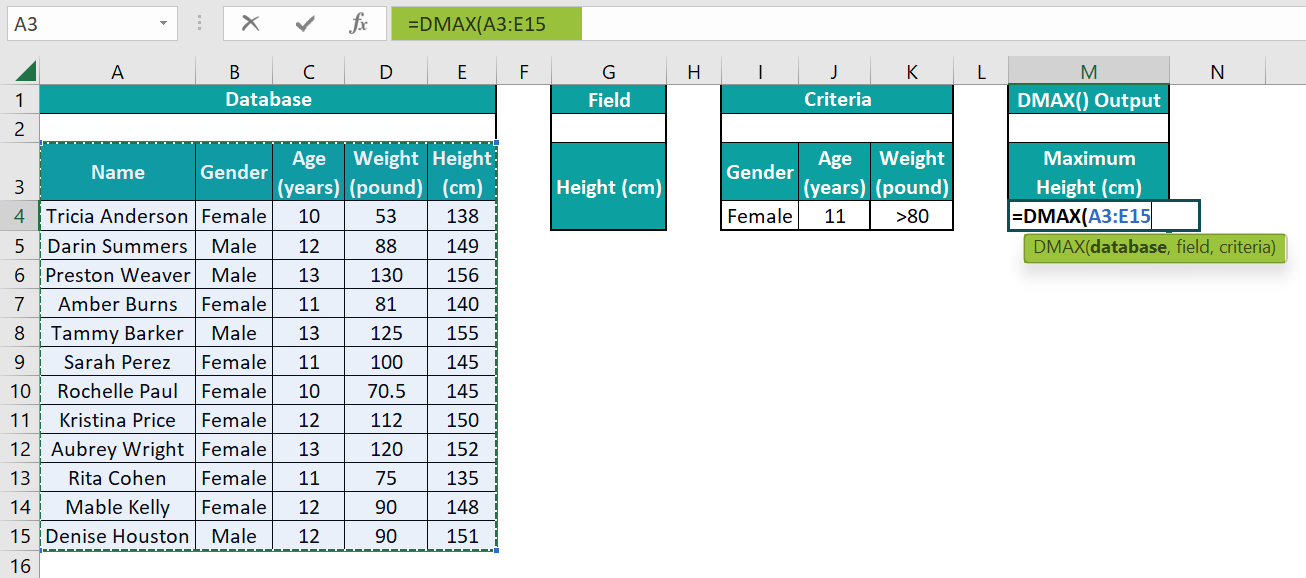2. Enter the field value as “Height (cm)”. The formula now is =DMAX(A3:E15,”Height (cm)”,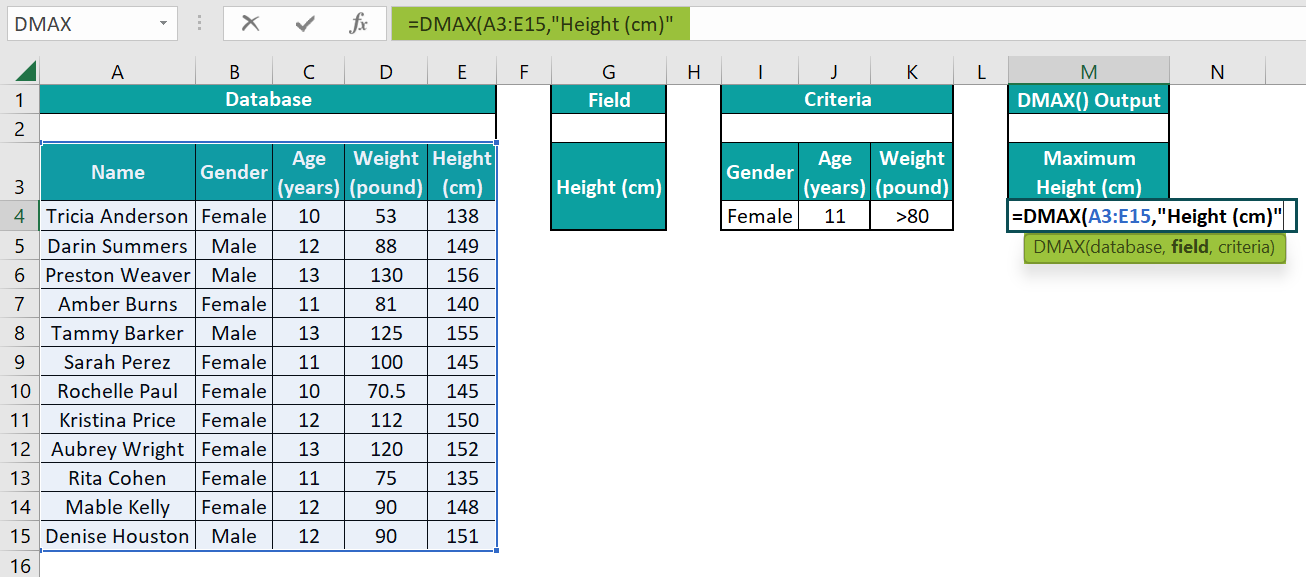3. Enter the criteria as “I3:K4”, close the brackets, and press “Enter”. The complete formula =DMAX(A3:E15,”Height (cm)”,I3:K4). The result is 145, as shown below.

[Note: Alternate formulas are =DMAX(A3:E15,5,I3:K4) or =DMAX(A3:E15,G3,I3:K4)]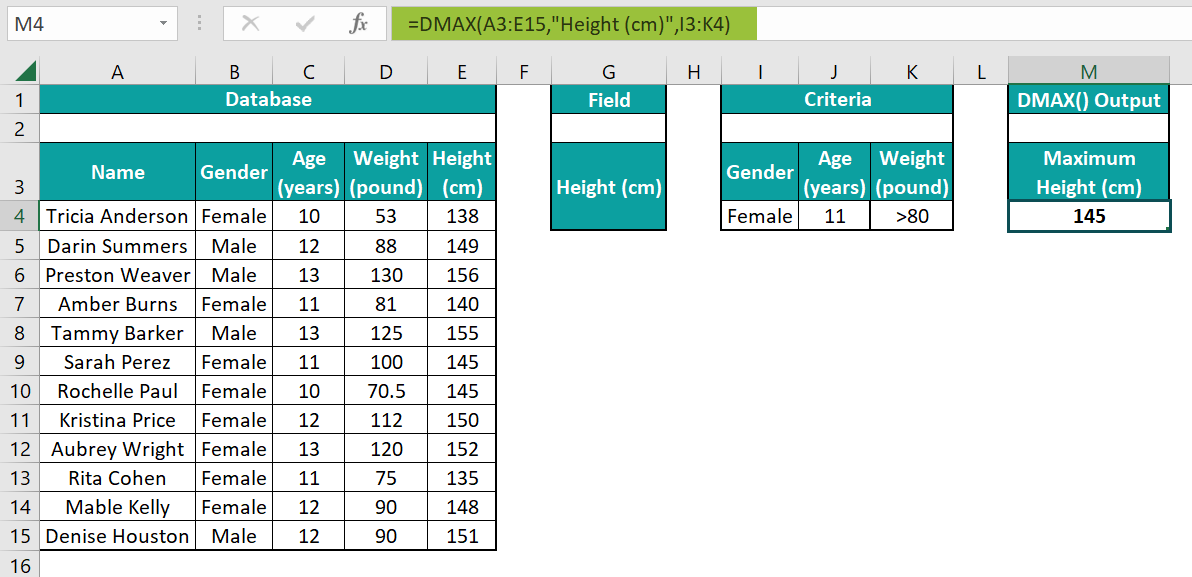[Alternatively, select cell M4 and Homefx icon or press Shift + F3 to open the Insert Function window.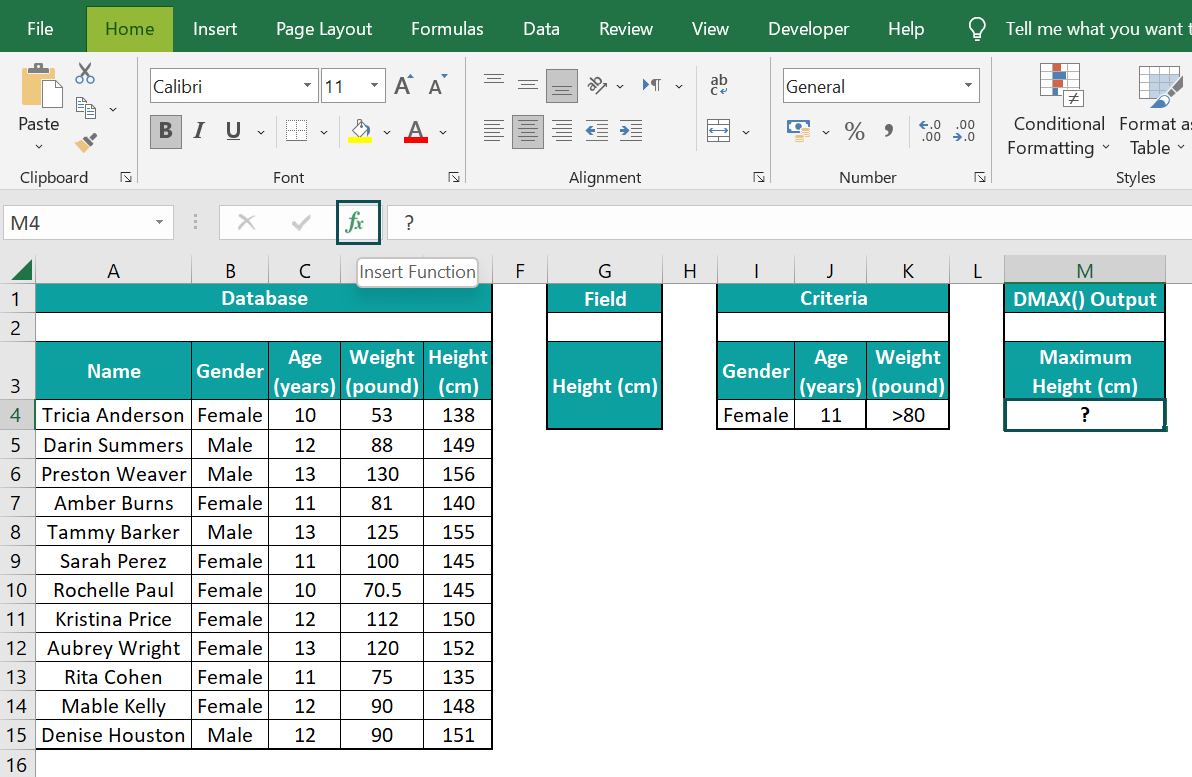Otherwise, navigate FormulasInsert Function to open the Insert Function window.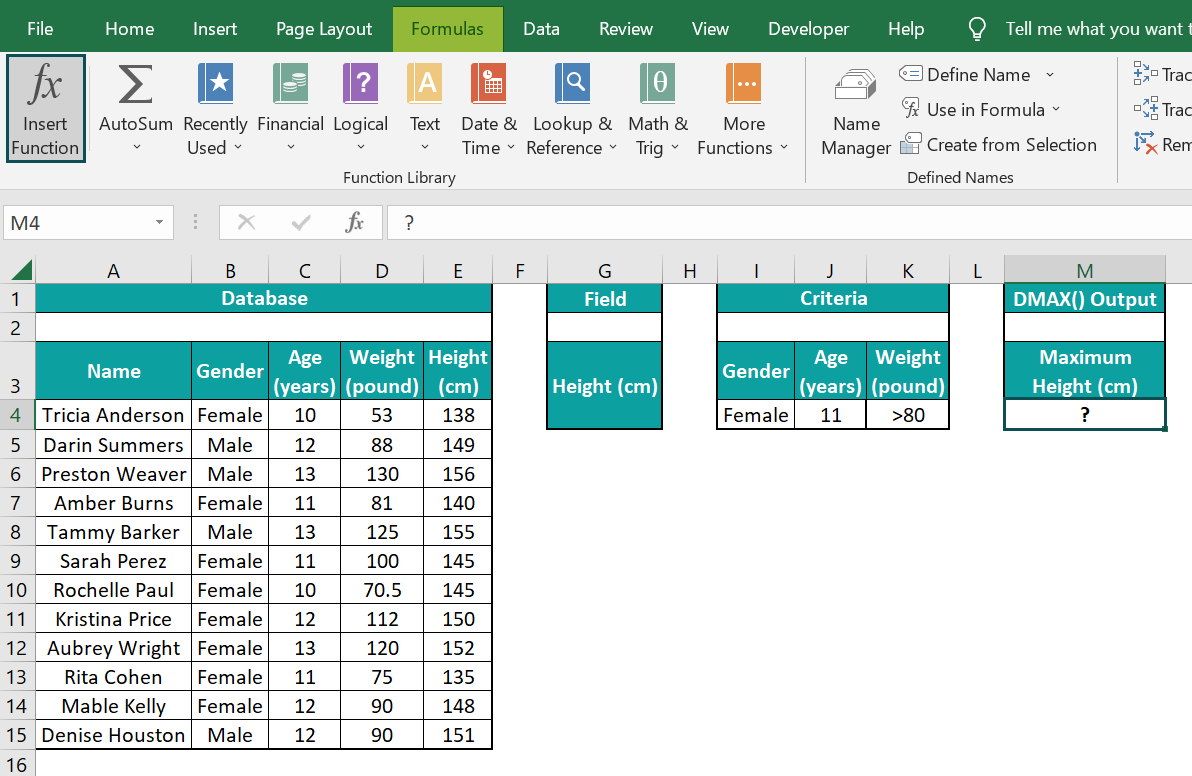The “Insert Function” window appears.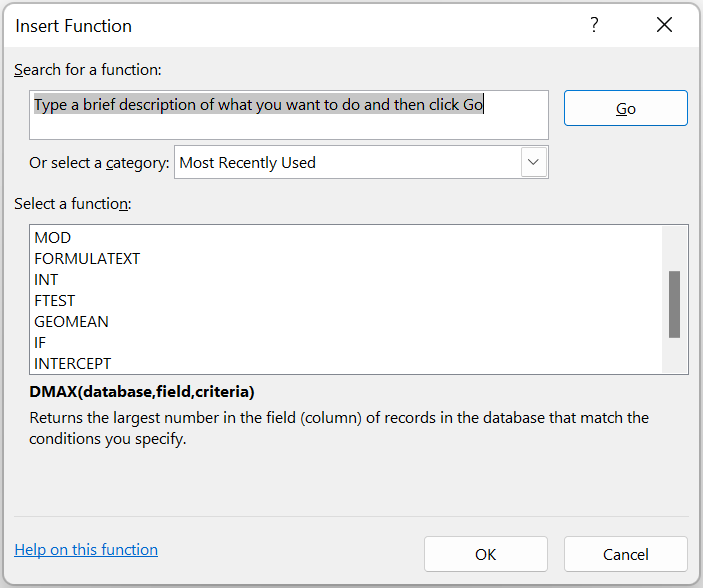Next, select the category as Database, select the DMAX function, and click OK.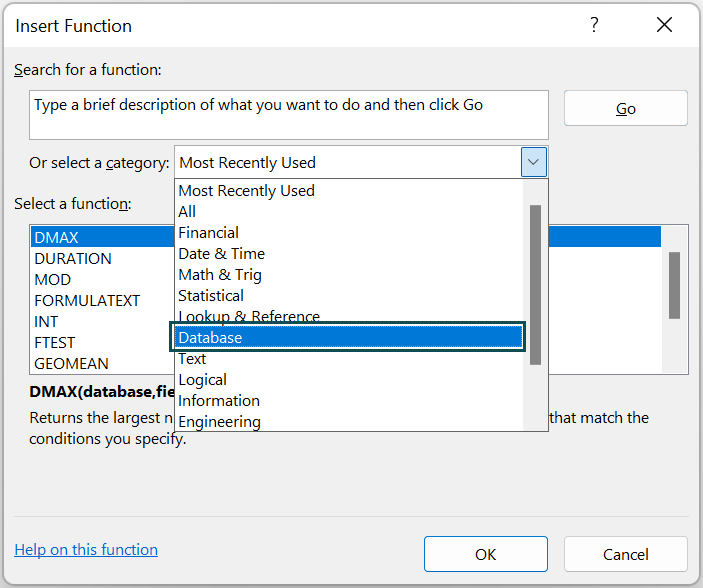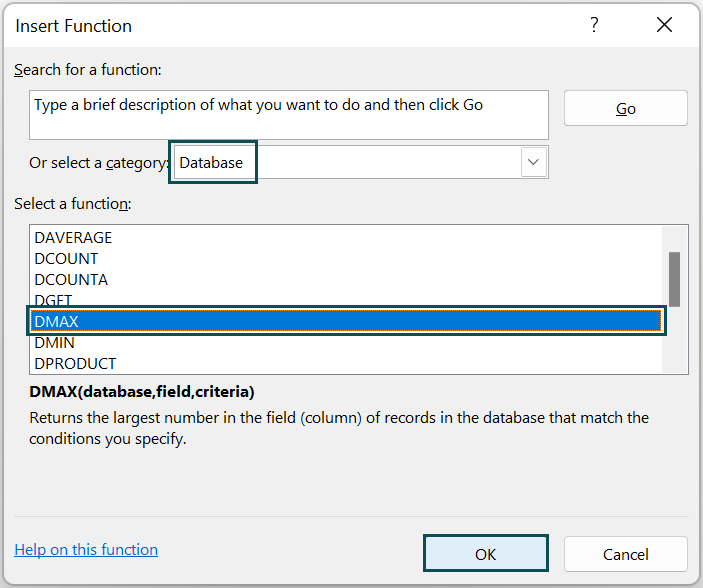The above steps will open the Function Arguments window. Now, enter the three argument values as shown below.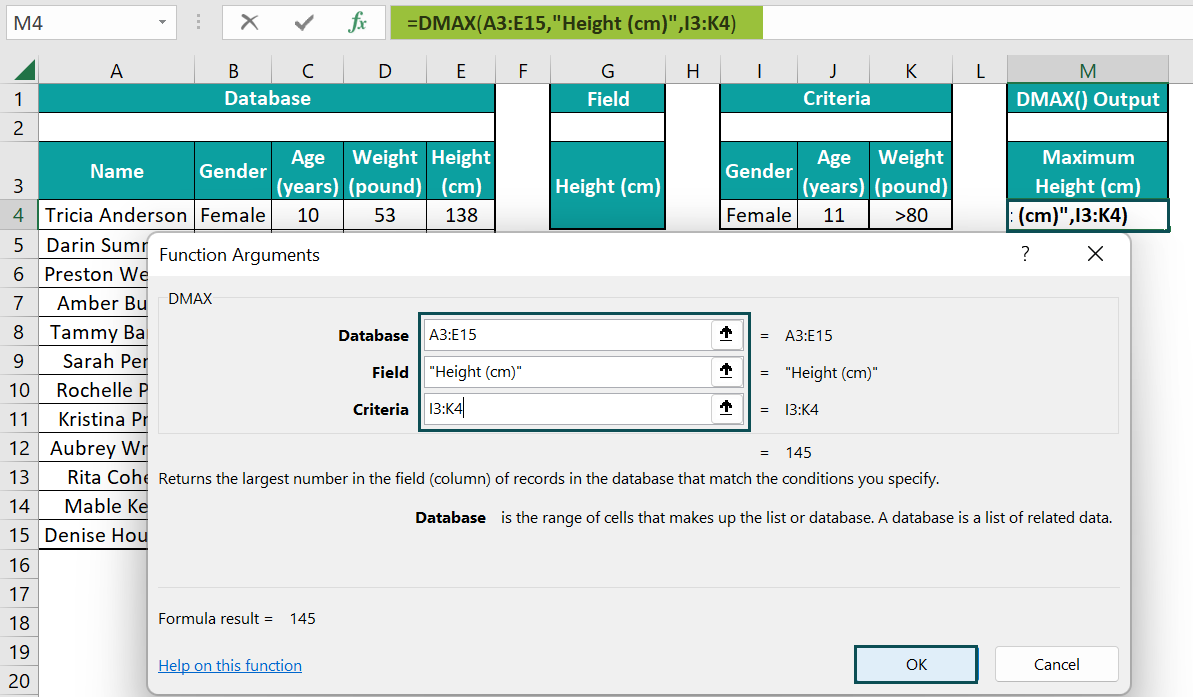And once we click OK, we will see the DMAX() executed in the target cell M4.]

[Output Observation: In cell M4, the function checks the database for the female gender, age 11, and weight values greater than 80 pounds. Two rows, 7 and 9, satisfy the specified criteria. And out of the two rows, row 9 has a higher height value in cell E9, 145. And thus, the DMAX() returns 145 cm as the required maximum height value.]

### Examples

We will understand some advanced scenarios using the DMAX in Excel examples.

#### Example #1

We will determine the maximum total order cost for a Dell laptop with an ordered quantity greater than ten and whose cost per unit is over \$1,000 for the following data.

• The first table in the below image is a database containing laptops’ order data.
• Next, the second one shows the field to check for the required maximum total order cost.
• The third table contains the criteria to decide the highest value.
• Also, the data format of the target cell H10 is Currency (highlighted in the image below), as the DMAX() output is a currency value. We will also see how to use a named range as a function input.

The steps to calculate using the DMAX() are,

• Step 1: Select the cell range A3:E18 and go to FormulasDefine Name to open the New Name window.
• Step 2: Enter the name in the New Name window we require to assign to the selected cell range and click OK to close the window.
• Step 3: Select the target cell H10, and enter the DMAX formula in the following way.

Once we start typing the formula and enter the database cell range name, Excel will show the complete defined name for us to select.

Double-click the defined name, enter a comma, and then continue entering the remaining formula as =DMAX(Laptop_Order_Data,”Total Order Cost in USD”,I3:K4)

• Step 4: Press Enter to view the DMAX() output. The result is ‘\$23,199.84’, as shown below.

[Output Observation: The DMAX() accepts the database argument as a named range, Laptop_Order_Data. The field argument value is “Total Order Cost in USD”, and the criteria argument value is the cell range I3:K4.

Rows 12 and 16 meet the criteria, where the total order costs are \$21,749.85 and \$23,199.84. And out of the two, as row 16 has a higher total order cost, the DMAX() output is \$23,199.84.]

#### Example #2

We will determine the maximum popularity value among the iPhone models with fame greater than 80% in the given database. The example also explains how to use the wildcard characters in DMAX in Excel.

• The first table shows a database containing a list of smartphones, their fame, and popularity details.
• Also, the output is a percentage value, we will set the target cell data format as Percentage (highlighted in the image below).

The procedure to calculate the DMAX is,

Select cell J4, enter the formula=DMAX(A3:C11,”Popularity in %”,G3:H4), and press Enter.

The result is ‘48%’, as shown below.

[Output Observation: The Smartphone criterion contains the wildcard character ‘*’, followed by the term, Phone. So, the DMAX() checks the database for smartphone models containing the term Phone in their names. Thus, it counts iPhone models with fame greater than 80%, which is the second criterion.

Rows 7 and 11 satisfy the criteria, where the popularity values are 48% and 39%. And, out of the two, the DMAX() returns the larger value, 48%, as the maximum popularity.]

#### Example #3

We will now see how the function DMAX in Excel works when the criteria argument is the entire database. We will determine the maximum population value based on the given database.

• The first table shows a database containing US cities’ population data.
• Next, the second includes the field argument to check for the maximum population.
• The third one indicates that the entire database is the criteria.
• Also, the target cell J4 data format is Number (highlighted in the below image), the same as that of cells C4:C13.

The procedure to calculate the DMAX is,

Select cell J4, enter the formula =DMAX(A3:C13,”Population”,A3:C13), and press Enter.

The result is ‘8,804,190’, as shown below.

[Output Observation: The DMAX() checks the entire database and looks for the record containing the maximum population in the Population field. Here, the criteria argument is the entire database. And hence we provide the criteria cell range is the same as the database cell range.

And, as row 8 meets the given criteria, the DMAX function returns the cell C8 population value, 8,804,190, as the required output.]

### Important Things To Note

• If the supplied field argument in DMAX in Excel is a column label, we can supply it directly in double quotes or as a cell reference to the column label. Otherwise, we can enter the value as the specific column position in the database.
• Ensure the supplied database and criteria cell ranges contain the same column labels.
• Ensure the criteria cell range contains at least one column label and a cell containing the required criterion below it. And to operate on an entire column in the given database, provide a blank line after the column labels in the criteria cell range.
• While the term record refers to a row of related information, the term field indicates a column of data in a database.

1. Is there a DMAX function in Excel?

The DMAX function in Excel is available in the Database function in the Formulas tab.
We can go to FormulasInsert Function to open the Insert Function window.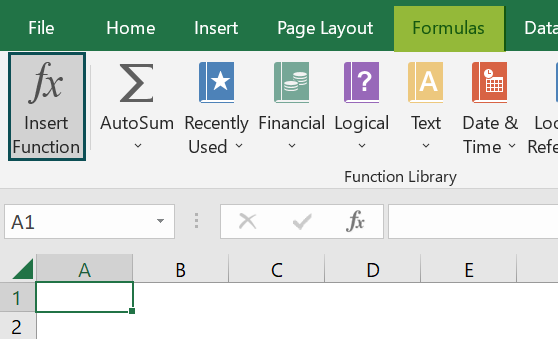Otherwise, click the fx option in the Home tab to open the Insert Function window.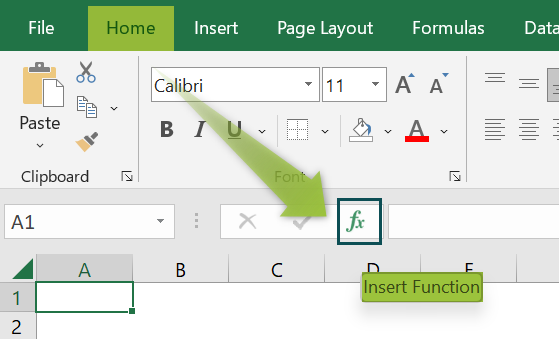Next, set the category as Database, pick the DMAX function, and click OK to apply it in the selected cell.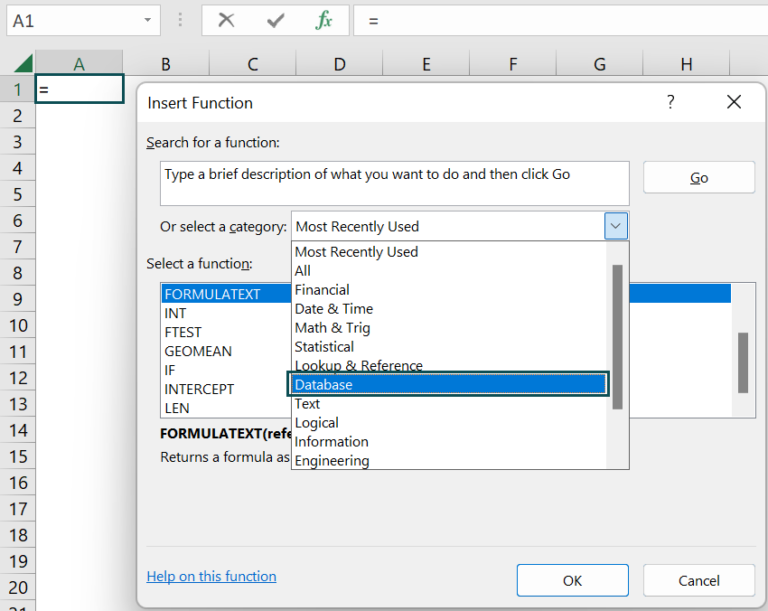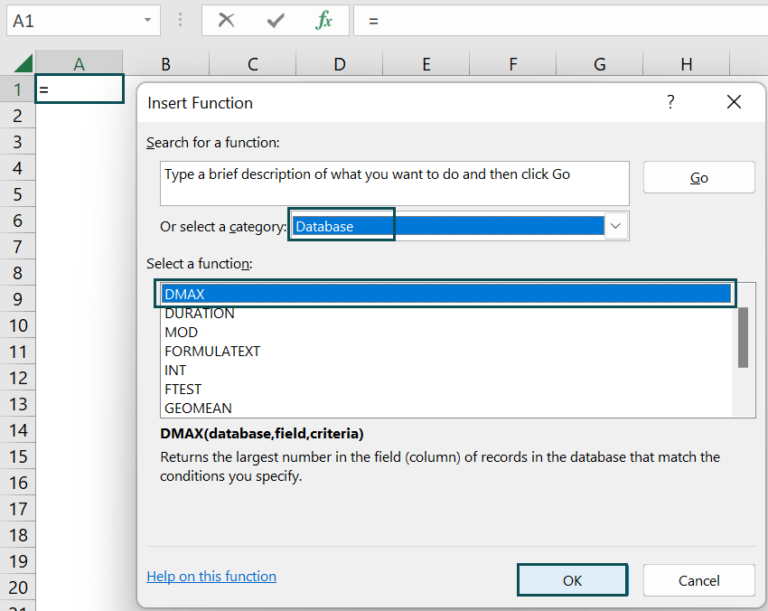2. How to apply the DMAX function in Excel VBA?

We can apply the DMAX function in Excel VBA using the following method:

Application.WorksheetFunction.DMax(Arg1, Arg2, Arg3)

The three arguments in the above method are the arguments, database, field, and criteria explained in the DMAX() Excel Formula section.

We will determine the maximum duration needed for completing a March month task requiring below 20 FTEs using the DMAX() and Excel VBA for the following data.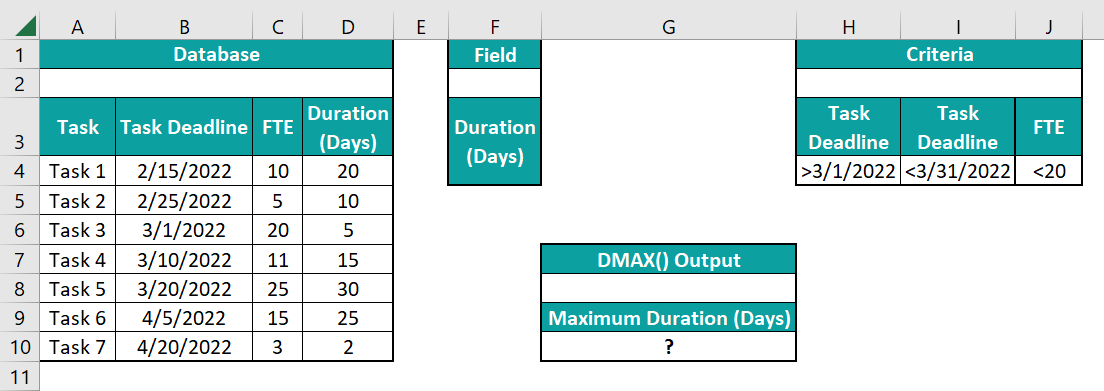The steps to apply the DMAX function in VBA are,

• 1: With the current sheet open, use the Alt + F11 keys to open the VBA Editor.• 2: Pick the required VBAProject and select the Module option under the Insert tab to open a module window, Module1.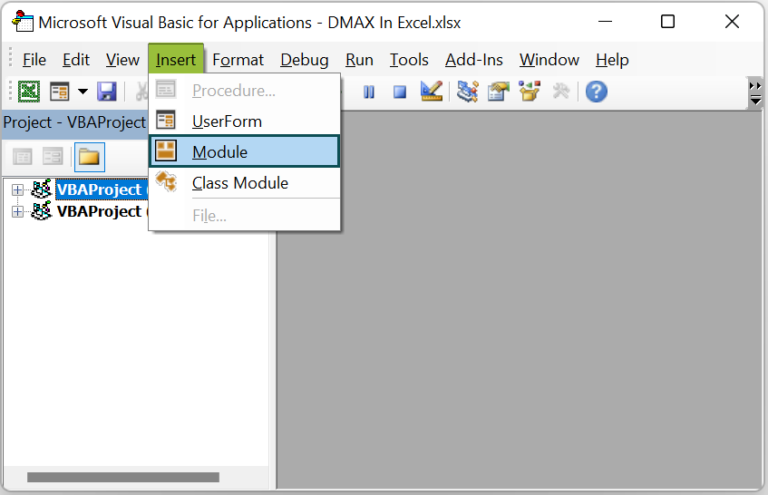• 3: Enter the VBA code, shown below, to apply the DMAX() in the target cell.

Sub DMAX_fn()

Dim ws As Worksheet
Set ws = Worksheets(“FAQ_2”)

ws.Range(“G10”) = Application.WorksheetFunction.DMax(ws.Range(“A3:D10”), “Duration (Days)”, ws.Range(“H3:J4”))

End Sub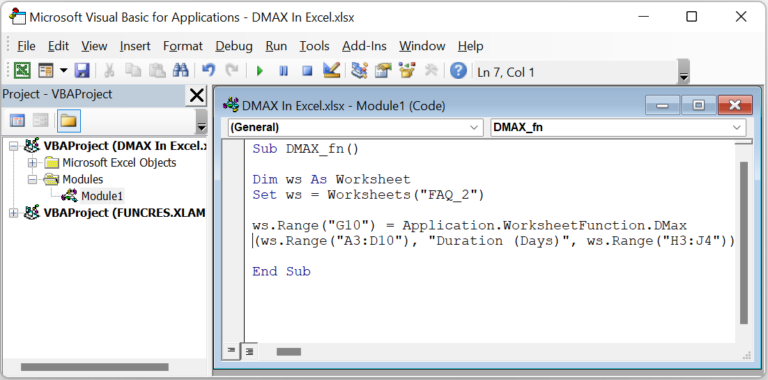• 4: Execute the code using the Run Sub/UserForm option in the top menu.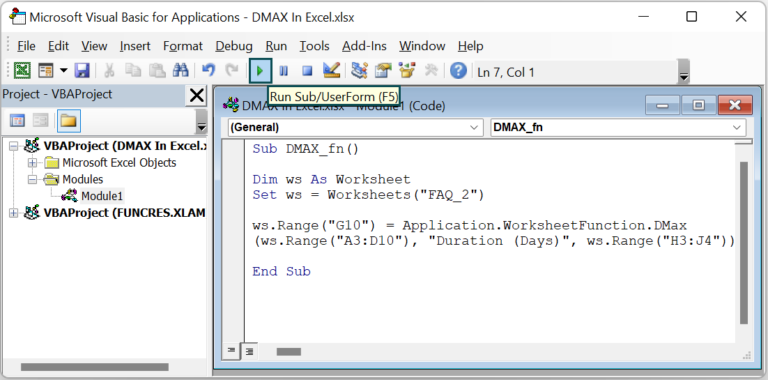• 5: Open the active worksheet to check the target cell G10 for the required DMAX() output.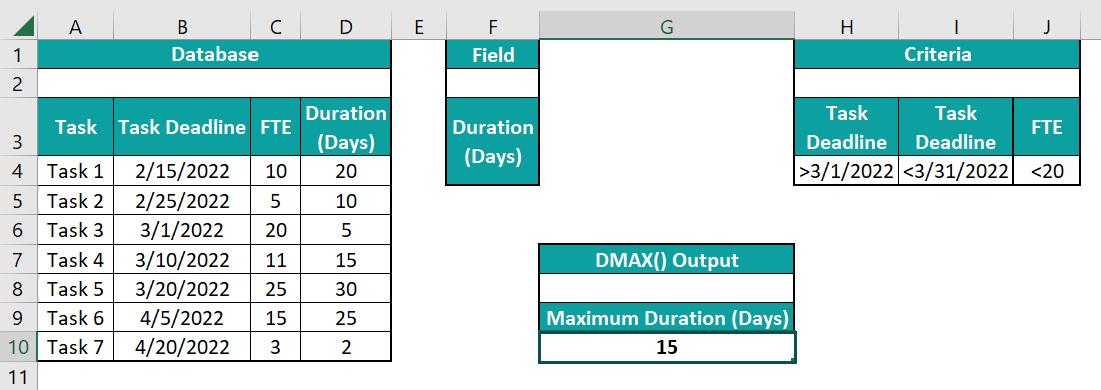[Output Observation: The DMAX() checks the dates in the database falling in March, with the FTEs count less than 20. And as only row 7 meets the criteria, the DMAX function returns the duration value in cell D7, 15, as the required maximum duration.]

3. Why is the DMAX function not working in Excel?

The DMAX function might not work for the following reasons:
If the specified field to check for the largest value does not contain numeric values.
The directly supplied column label as the field argument is not in double quotations, or the provided column position is incorrect.
If the criteria argument is other than numeric values, text, or expressions.
The provided database and criteria cell ranges do not include the same column labels.
The specified database and criteria cell ranges overlap.
When an operation involves an entire column in the given database, we do not enter a blank line after the column labels in the criteria cell range.